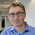## Saturday, June 9, 2012

### Rcpp vs. R implementation of cosine similarity

While speeding up some code the other day working on a project with a colleague I ended up trying Rcpp for the first time. I re-implemented the cosine distance function using RcppArmadillo relatively easily using bits and pieces of code I found scattered around the web. But the speed increase was not as much as I expected comparing the Rcpp code to pure R.
And here is the speed comparison...

I don't know really if my implementation can be improved? For example, there is this step at the beginning where the R matrix is transformed to a Rcpp::NumericMatrix and then to an arma::mat matrix. I could not ran the code without this step. I don't think it plays that much into the run time anyway as it should be all in-memory operation but I would be curious to know if there is another way.

#### 4 comments:

1.Not sure if it will be faster but you can create X using `arma::mat X = Rcpp::as< arma::mat >(Xs);`

2.If you use the byte compiler for the cosine (native R) function, you may see improvements in the speed there as well.

?compile
or
http://stat.ethz.ch/R-manual/R-devel/library/compiler/html/compile.html

3.Thanks for the suggestions. I did try the compiled version of cosine but saw no difference with native R and ended up not posting it. Here is a more complete benchmark:
cosineRcpp2 follow @Jeffrey suggestion and cosC = cmpfun(cosine).

res
test replications elapsed relative user.self sys.self
2 cosineRcpp(mat) 100 15.749 1.000000 13.925 0.771
3 cosC(mat) 100 16.460 1.045146 14.587 1.075
4 cosineRcpp2(mat) 100 16.595 1.053718 14.090 0.785
1 cosine(mat) 100 17.032 1.081465 14.767 1.075

4.For the native R code try getting rid of the brackets and posting the steps sequentially. R brackets slow down the function quite a bit....
for instance:
"res <- 1 - y / (sqrt(diag(y)) %*% t(sqrt(diag(y))))"
can be:
"res.a <- diag(y)
res.a <- sqrt(res.a)
res.b <- t(res.a)
res <- res.a %*% res.b
res <- y/res
res <- 1 - res"